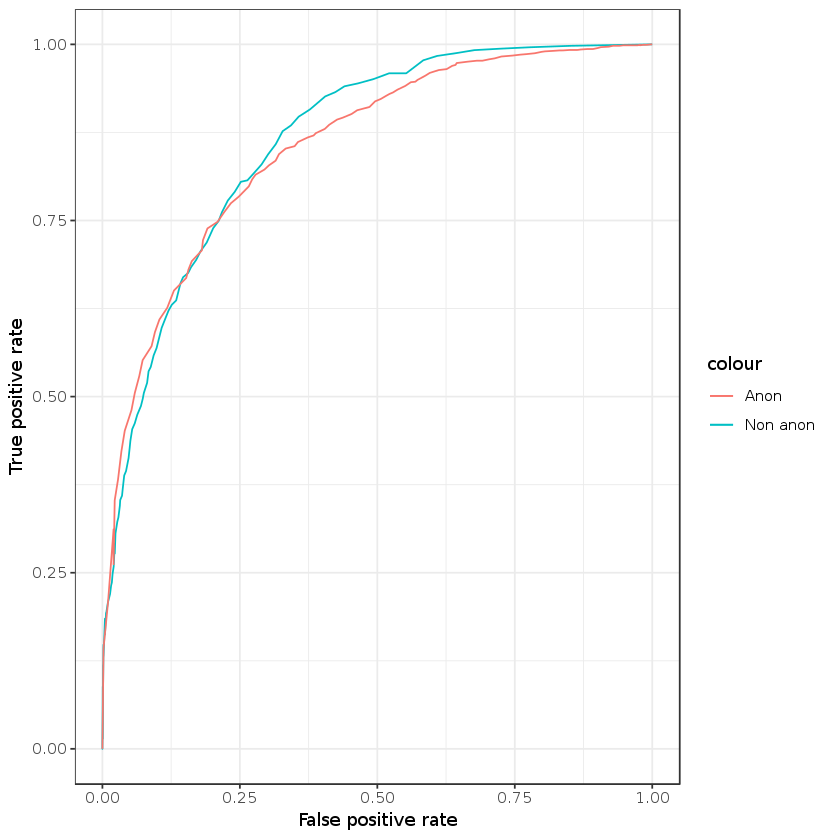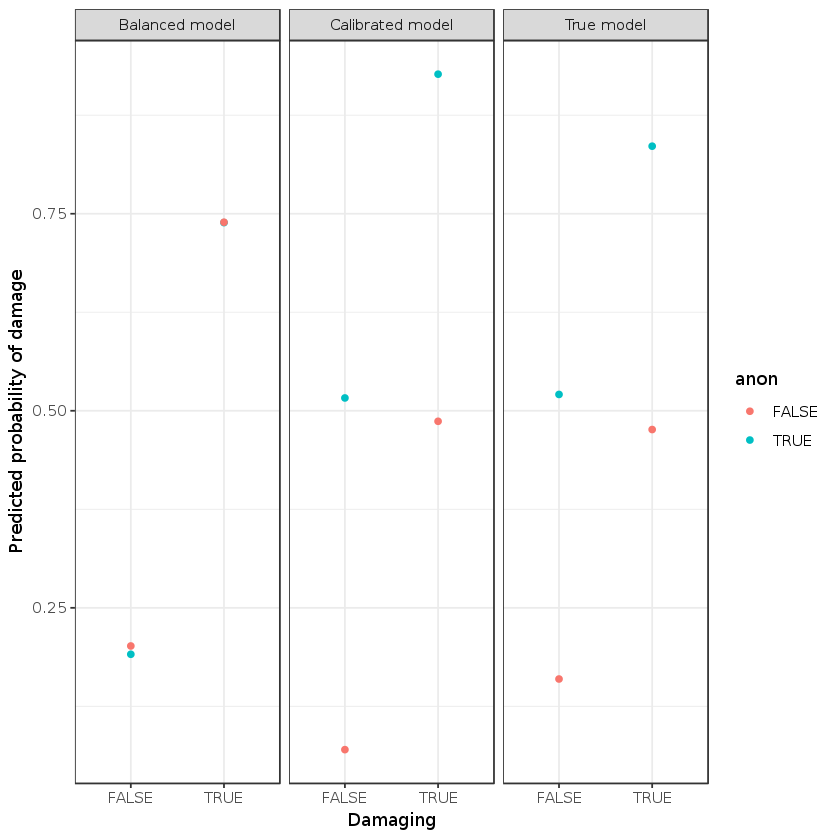# Illustrating the tradeoff between balance and calibration¶

## using the example of anonymous Wikipedia editors¶

I wrote here about the bias encoded into the ORES models deployed on Wikipedia for helping editors to monitor changes to the encyclopedia. There I showed that the models were unfair to newcomers and anonymous editors using two different notions of fairness: balance and calibration. I brought up the fact that there is an inherent tradeoff between these two quantified notions of fairness such that in non-trivial situations it is impossible to satisfy them both. Here, I’m going to illustrate this point with a simple simulation and show how a straightfoward approach to creating a balanced model from an imbalanced one results in a model which is not calibrated.

In :
# I'm going to use these R packages
library(ggplot2)
theme_set(theme_bw())
library(data.table)


Let’s say that whether an edit is damaging is a stochastic function of two observable variables: whether the editor is anonymous and X, which stands for everything else we can observe and include in our model. We’ll say the linear probit model with these two variables is the true model.

In :
# generate a dataset according to the model
B_anon <- 2
B_X <- 1
n <- 4000
edits <- data.table(anon=c(rep(TRUE,n/2),rep(FALSE,n/2)), X = rnorm(n/2,0,1))
edits[,p_damaging := pnorm(B_anon*anon + B_X*X,1,1)]
edits[,damaging := sapply(p_damaging, function(p) rbinom(1,1,p))]


Next I’ll fit a model to the generated data and generate model predictions

In :
glm_mod = glm(damaging ~ anon + X - 1, data = edits,family=binomial(link='probit'))
edits[,p.calibration := pnorm(predict(glm_mod,newdata=edits))]
edits[,calibration.pred:= p.calibration > 0.5]


The true model should be calibrated, but not balanced. Let’s verify that is the case.

In :
edits[, .(model=mean(p.calibration), true=mean(damaging)),by=c("anon")]

anonmodeltrue
TRUE 0.75345750.7540
FALSE 0.24292440.2435

So we see that it is calibrated, but is it balanced?

In :
edits[,mean(p.calibration),by=c("damaging","anon")]

damaginganonV1
0 TRUE 0.5044010
1 TRUE 0.8347147
0 FALSE 0.1619012
1 FALSE 0.4946453

Not even close! The model is super unbalanced. Non-damaging anonymous edits have almost the same average score as damaging non-anonymous edits!

Some people think that you can sovle algorithmic bias problems by using feature engineering and ignoring protected classes. There are some merits to this approach, but it isn’t a great solution to the balance vs calibration tradeoff. To illustrate this point, let’s fit another model that only uses X and ignores anons.

In :
glm_mod2 = glm(damaging ~  X , data = edits,family=binomial(link='probit'))
edits[,p.try_balance := pnorm(predict(glm_mod2,newdata=edits))]
edits[,mean(p.try_balance),by=c("damaging","anon")]

damaginganonV1
0 TRUE 0.2828035
1 TRUE 0.5694571
0 FALSE 0.4274849
1 FALSE 0.7209365

The model is still imbalanced! But that did seem to make things a little bit better. Is the model still calibrated?

In :
edits[, .(model=mean(p.balance1), true=mean(damaging)),by=c("anon")]

anonmodeltrue
TRUE 0.49894030.7540
FALSE 0.49894030.2435

No it’s really not calibrated now! So ignoring anons makes a choice about the tradeoff between balance and calibration, but it does so in an arbitrary way that depends on myriad factors including the correlation between anonymous editing and X.

A better approach to creating a balanced model comes from Hardt et al. (2016). Since the point where the ROC curves for the two protected classes intersect corresponds to choices of threshholds with equal false positve and negative rates, you can transform a good predictor to a worse predictor that is balanced by using different threshholds for different types of editors.

Plot the ROC curves.

In :
roc_x <- 0:100/100
tpr_anon <- edits[anon==TRUE & damaging == TRUE, sapply(roc_x, function(x) mean(p.calibration > x))]
fpr_anon <- edits[anon==TRUE & damaging == FALSE, sapply(roc_x, function(x) mean(p.calibration > x))]
tpr_nonanon <- edits[anon==FALSE & damaging == TRUE, sapply(roc_x, function(x) mean(p.calibration > x))]
fpr_nonanon <- edits[anon==FALSE & damaging == FALSE, sapply(roc_x, function(x) mean(p.calibration > x))]
roc <- data.table(x=roc_x,tpr_anon=tpr_anon,fpr_anon=fpr_anon,tpr_nonanon=tpr_nonanon, fpr_nonanon=fpr_nonanon)
ggplot(roc) + geom_line(aes(x=fpr_nonanon,y=tpr_nonanon,color="Non anon")) + geom_line(aes(x=fpr_anon,y=tpr_anon,color="Anon")) + ylab("True positive rate") + xlab("False positive rate")So it looks like we can find balance with the FPR is around 0.2. We see below that we have quite dramatically shifted the threshholds in favor of anons.

In :
(t.nonanon <- roc_x[which.min(abs(fpr_nonanon - 0.2))])

0.29
In :
(t.anon <- roc_x[which.min(abs(fpr_anon - 0.2))])

0.77

Let’s make new predictions and check balance and calibration. Note that now our threshhold for classifying an edit as damaging is much higher for anons than for non-anons.

In :
## for anons its where fpr_anon is about 0.22 which is at about 0.77
## you can use linear programming to do this but i'm lazy
edits[anon==TRUE, balance.pred := p.calibration > t.anon]
edits[anon==FALSE, balance.pred := p.calibration > t.nonanon]
edits[,mean(balance.pred),by=.(damaging,anon)]

damaginganonV1
0 TRUE 0.1910569
1 TRUE 0.7387268
0 FALSE 0.2015863
1 FALSE 0.7392197

Using different threshholds for the different classes gives us a nearly balanced classifier!
The next question is if the balanced predictor is calibrated. What do you expect?

In :
## check if the classifier is calibrated. No way!
edits[,.(Predicted=mean(balance.pred), True=mean(damaging)), by=c("anon")]

anonPredictedTrue
TRUE 0.60400.7540
FALSE 0.33250.2435

Nope! Not balanced. The predicted rate of vandalism for anons is lower than the true rate and for non-anons the predicted rate of vandalism is greater than the true rate. Finally, we can visualize the difference between calibration and balance.

In :
balance.rates = edits[,mean(balance.pred),by=c('damaging','anon')]
balance.rates[,level := 'Balanced model']
calibration.rates = edits[,mean(calibration.pred),by=c('damaging','anon')]
calibration.rates[,level:='Calibrated model']
true.rates = edits[,mean(p_damaging),by=c('damaging','anon')]
true.rates[,level:='True model']
dt <- rbind(balance.rates, calibration.rates, true.rates)
ggplot(dt,aes(x=damaging==TRUE,color=anon,group=anon,y=V1)) + geom_point() + facet_wrap(.~level) + xlab("Damaging") + ylab("Predicted probability of damage")How much accuracy did we lose by making our model balanced? Of course, this will depend on the particulars of how I simulated the data.

In :
(acc_calib <- edits[,mean(calibration.pred == (damaging==TRUE))])
(acc_trybal <- edits[,mean((p.try_balance > 0.5) == (damaging==TRUE))])
(acc_bal <- edits[,mean(balance.pred == (damaging == TRUE))])

0.82
0.69225
0.77

Even though in this simulated data anons were three times as likely to make damaging edits compared to non-anons, balancing the model only costs 5 percentage points of accuracy. Moreover, the balanced model has better accuracy than the model that ignores that anons exist!

As a final point, observe that choosing the point where the ROC curves for the two groups intersects is a good way to choose threshholds that will balance the model, but this comes at the cost of calibration. Removing the anon variable from the model is a way to compromise between balance and fairness, but potentially at the cost of accuracy (in this exercise, the cost in accuracy was quite high, but if we increase the rate of X enough it will not matter much). However, Wikipedians might want to make a compromise between balance and fairness in a more principled way. One way to do this would just be to choose different threshholds for anons and for non-anons that may not accomplish total balance, but that preserve more calibration. There are also good approaches based on adding constraints to the model that carefully penalize deviations from balance and calibration,